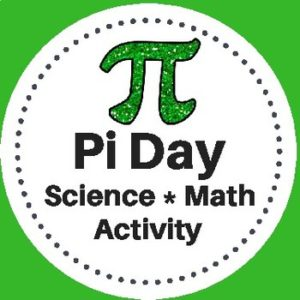Showing all 2 results

•\$3.00

This Pi Day Activity has been designed for Science and Math classrooms. Students will use measuring tools to determine the radius, diameter, and circumference of various circular objects. They will then use the data that they collect to calculate pi.

*This activity gives students the opportunity to clearly understand the concept of the number pi and practice math skills through a hands-on activity with a variety of circular objects.

•\$9.99

A step by step Geometry curriculum to teach students with ease. Students will learn about angles, lines, triangles, polygons,quadrilaterals, prisms, coordinate geometry, slope and more!